# Plotting opposite integers on a number line Online Quiz

#### Complete Python Prime Pack

9 Courses     2 eBooks

#### Artificial Intelligence & Machine Learning Prime Pack

6 Courses     1 eBooks

#### Java Prime Pack

9 Courses     2 eBooks

Following quiz provides Multiple Choice Questions (MCQs) related to Plotting opposite integers on a number line. You will have to read all the given answers and click over the correct answer. If you are not sure about the answer then you can check the answer using Show Answer button. You can use Next Quiz button to check new set of questions in the quiz.### Explanation

Step 1:

The opposite of 19 is −19.

Step 2:

These integers on number line are as shown below.### Explanation

Step 1:

The opposite of −23 is 23.

Step 2:

These integers on number line are as shown below.### Explanation

Step 1:

The opposite of 32 is −32.

Step 2:

These integers on number line are as shown below.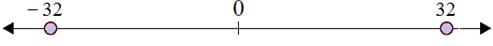### Explanation

Step 1:

The opposite of −45 is 45.

Step 2:

These integers on number line are as shown below.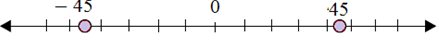### Explanation

Step 1:

The opposite of 77 is −77.

Step 2:

These integers on number line are as shown below.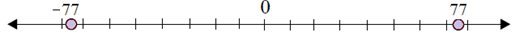### Explanation

Step 1:

The opposite of −15 is 15.

Step 2:

These integers on number line are as shown below.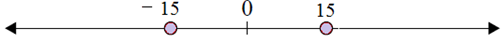### Explanation

Step 1:

The opposite of 27 is −27.

Step 2:

These integers on number line are as shown below.### Explanation

Step 1:

The opposite of −11 is 11.

Step 2:

These integers on number line are as shown below.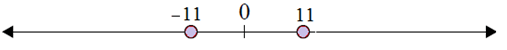### Explanation

Step 1:

The opposite of 55 is −55.

Step 2:

These integers on number line are as shown below.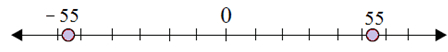### Explanation

Step 1:

The opposite of −38 is 38.

Step 2:

These integers on number line are as shown below.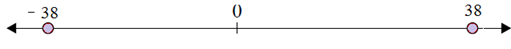plotting_opposite_integers_on_number_line.htm